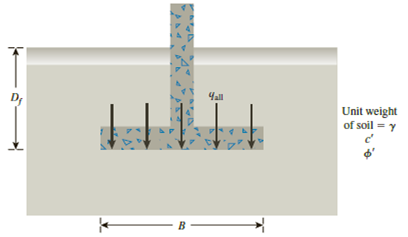Chapter 16, Problem 16.5PPrinciples of Geotechnical Enginee...

9th Edition
Braja M. Das + 1 other
ISBN: 9781305970939

Solutions

Chapter
SectionPrinciples of Geotechnical Enginee...

9th Edition
Braja M. Das + 1 other
ISBN: 9781305970939
Textbook Problem

Redo Problem 16.1 using the modified general ultimate bearing capacity Eq. (16.31).16.1 A continuous footing is shown in Figure 16.17. Using Terzaghi’s bearing capacity factors, determine the gross allowable load per unit area (qall) that the footing can carry. Assume general shear failure. Given: γ = 19 kN/m3, c′ = 31kN/m2, ϕ ′ = 28 ° , Df = 1.5 m, B = 2 m, and factor of safety = 3.5.Figure 16.17

To determine

The gross allowable load per unit area (qall) that the footing can carry using modified general ultimate bearing capacity.

Explanation

Given information:

The unit weight of the soil γ is 19kN/m3.

The value of cohesion c is 31kN/m2.

The soil friction angle ϕ is 28°.

The depth of foundation Df is 1.5 m.

The width of footing B is 2 m.

The factor of safety Fs is 3.5.

Calculation:

For a continuous foundation with vertical loading, all inclination factors and shape factors are equal to one.

Determine the depth factor λcd using the relation.

λcd=1+0.4(DfB)

Substitute 1.5 m for Df and 2 m for B.

λcd=1+0.4(1.52)=1.3

Determine the depth factor λqd using the relation.

λqd=1+2tanϕ(1sinϕ)2(DfB)

Substitute 28° for ϕ, 1.5 m for Df, and 2 m for B.

λqd=1+2tan28°(1sin28°)2(1.52)=1.224

Determine the ultimate bearing capacity of the soil (qu) using the relation.

qu=cNcλcd+qNqλqd+12γBNγλγd=cNcλcd+γDfNqλqd+12γBNγλγd (1)

Here, Nc is the contribution of cohesion, Nq is the contribution of surcharge, and Nγ is the contribution of unit weight of soil

Still sussing out bartleby?

Check out a sample textbook solution.

See a sample solution

The Solution to Your Study Problems

Bartleby provides explanations to thousands of textbook problems written by our experts, many with advanced degrees!

Get Started

Repeat Problem 2-1 without the possibility of rain accumulation on the roof.

Steel Design (Activate Learning with these NEW titles from Engineering!)

Who is ultimately responsible for the welder’s safety?

Welding: Principles and Applications (MindTap Course List)

Differentiate between a network attached storage (NAS) and a storage area network (SAN).

Enhanced Discovering Computers 2017 (Shelly Cashman Series) (MindTap Course List)